$\newcommand{\Indicator}{\mathbb{1}\left\{ #1 \right\}} \newcommand{\Prob}{\mathbb{P}\left( #1 \right)} \DeclareMathOperator{\sgn}{sgn}$

# Notation

$$\vec{X}$$ will be the $$p$$-dimensional vector of features we’re using for our predictions.

$$R$$ (for “radius”) will be the maximum magnitude of the $$n$$ training vectors, $$R \equiv \max_{1 \leq i \leq n}{\|\vec{x}_i\|}$$.

$$Y$$ will be the class we want to predict, usually by not always binary, $$0/1$$.

When we are dealing with binary classes, $$Z=2Y-1$$. That is $$Z=+1$$ when $$Y=1$$, but $$Z=-1$$ when $$Y=0$$. The $$Z$$ variable is of course redundant when we have $$Y$$, but it simplify a lot of the formulas.

$$\Indicator{A}$$ is $$1$$ when the expression $$A$$ is true, and $$0$$ otherwise. Similarly, $$\sgn{a}$$ is $$+1$$ when $$a > 0$$, $$-1$$ when $$a < 0$$, and $$0$$ when $$a=0$$.

# Linear classifiers (recap)

We say that we have a linear classifier, with feature-weights $$\vec{w}$$ and offset $$b$$, when we predict $\hat{Y}(\vec{x}_0) = \Indicator{b + \vec{w}\cdot\vec{x}_0 \geq 0}$ or equivalently $\hat{Z}(\vec{x}_0) = \sgn{\left(b+\vec{w}\cdot\vec{x}_0\right)}$ As usual, $$\vec{x}_0$$ is a totally arbitrary point in the feature space. It may or may not have been one of the training points, where we observed the corresponding class.

# We want to use many nonlinear features, but don’t actually want to calculate them all

We’ve seen some examples of building linear classifiers, and seen that they can work well. But there are also situations where they just don’t work at all. For example, looking at the data in the next figure1.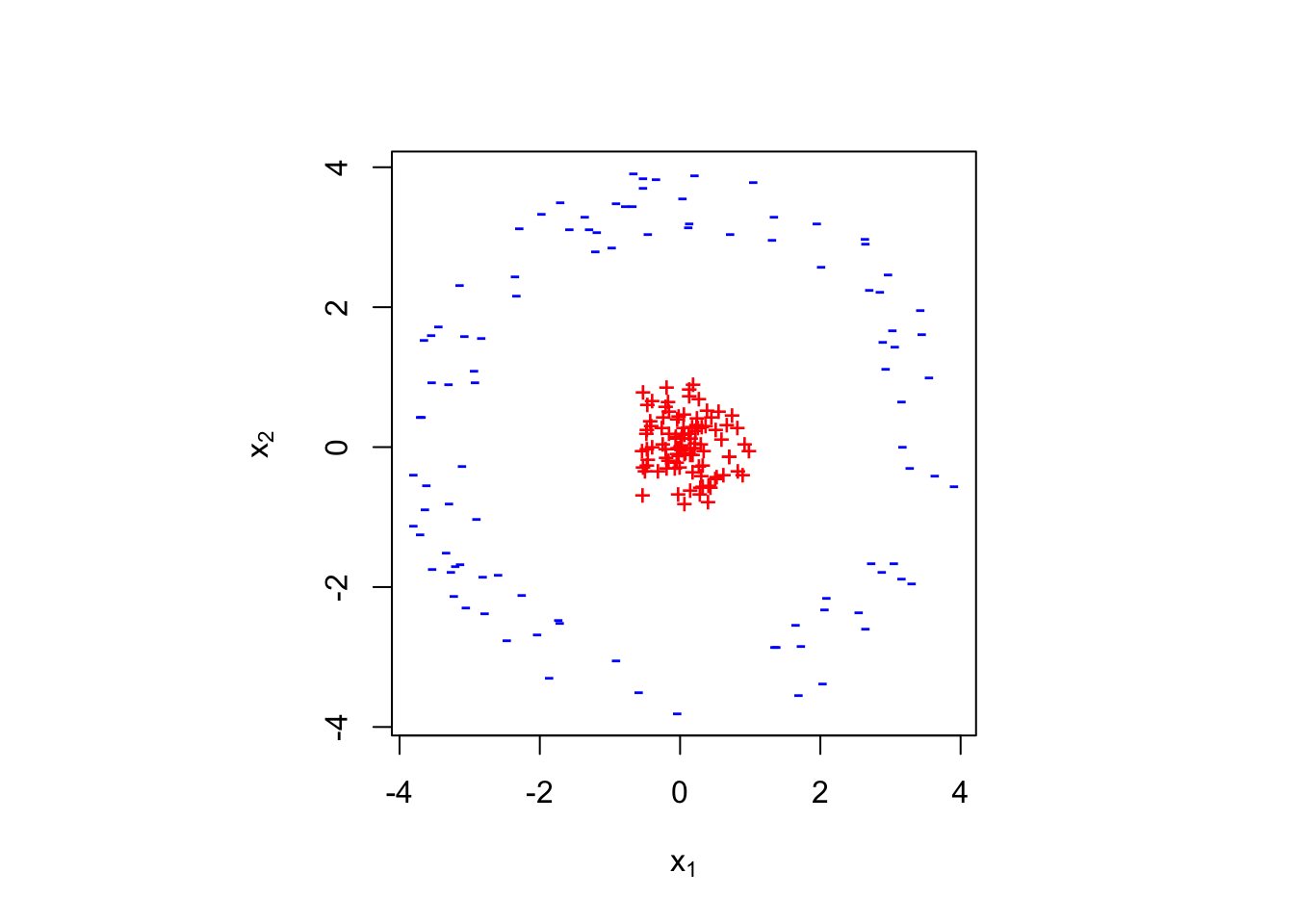If we use the given features $$x_1$$ and $$x_2$$, classifying points as $$+$$ or $$-$$ is a bit hard. There is no linear classifier here which will work perfectly.2 The prototype method will fail dismally here, doing no better than guessing at random3. You can find a line which does better than guessing at random4, but not much better.

Now of course separating these two classes is trivial if we can use a nonlinear boundary, like a circle: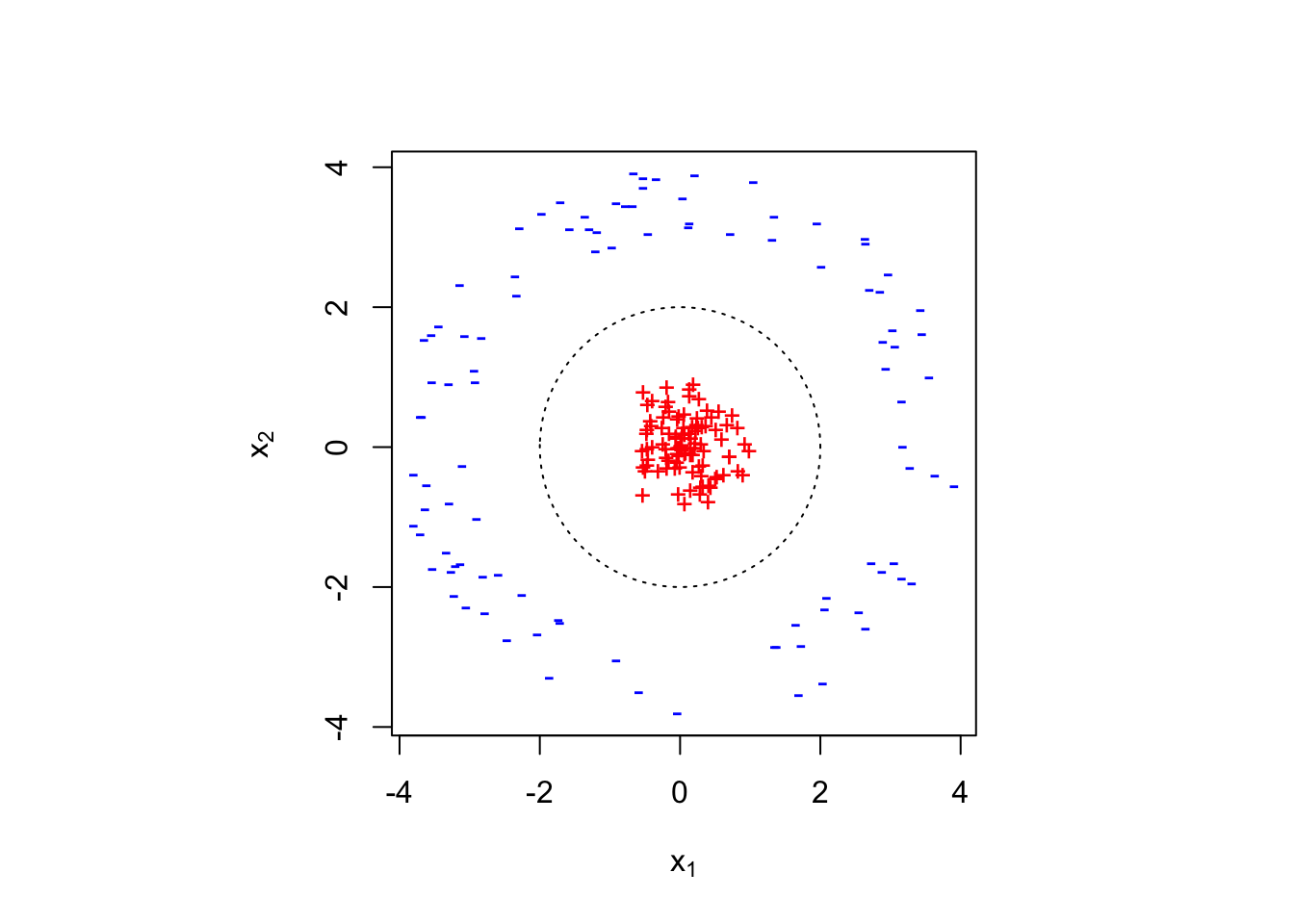We could start thinking about how we would start drawing such boundaries directly, but an alternative strategy has proved more useful. This is to transform the original features, nonlinearly, and then do linear classification in the new, transformed or derived features. To see how this can work, suppose we did the very simple thing of looking at the squares of the original features, $$x_1^2$$ and $$x_2^2$$.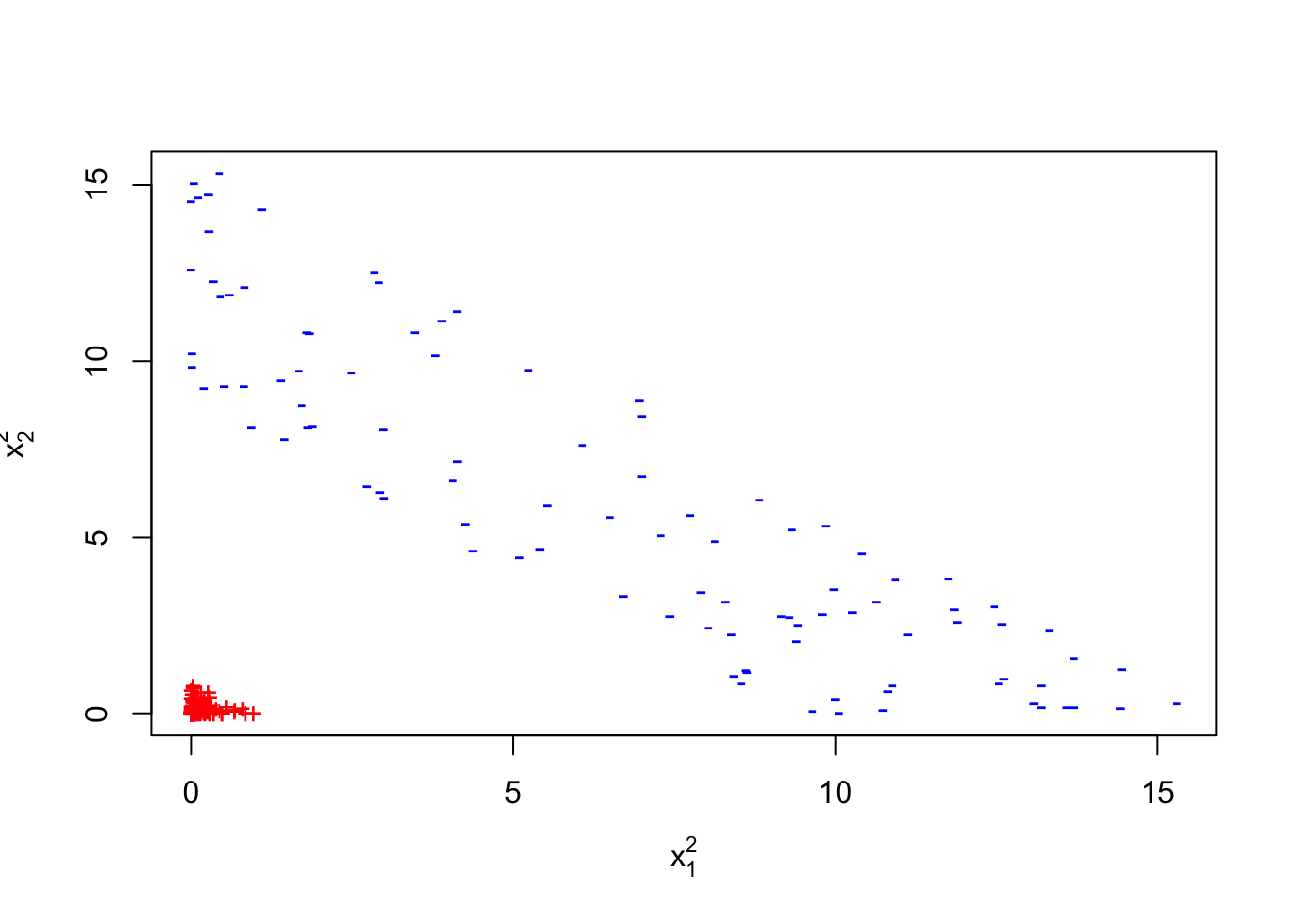(Of course, the squares of the original features aren’t the only derived features we could use. Switching to polar coordinates ( $$\rho = \sqrt{x_1^2 + x_2^2}$$ and $$\theta = \arctan{x_2/x_1}$$) would do just as nicely.)

In this example, we kept the number of features the same, but it’s often useful to create more new features than we had originally. This next figure shows a one-dimensional classification problem which also has no linear solution, since the class is negative if $$x$$ is below one threshold, or above another.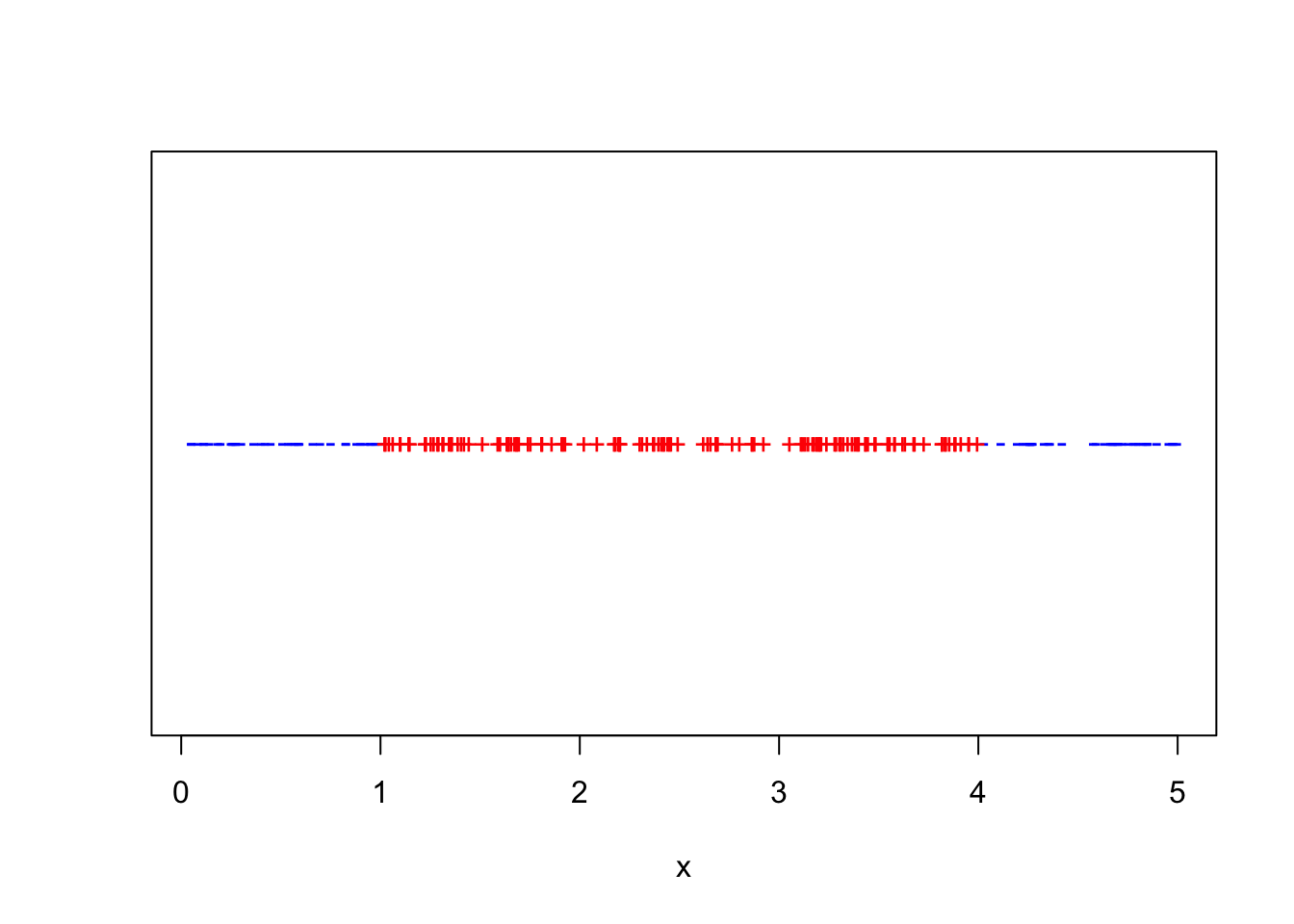Problems like this, where one of the original features must be either in one range or another (“exclusive-or” or “XOR” problems) cannot be solved exactly by any linear method5.

Adding a second feature like $$x^2$$ makes it easy to linearly separate the classes: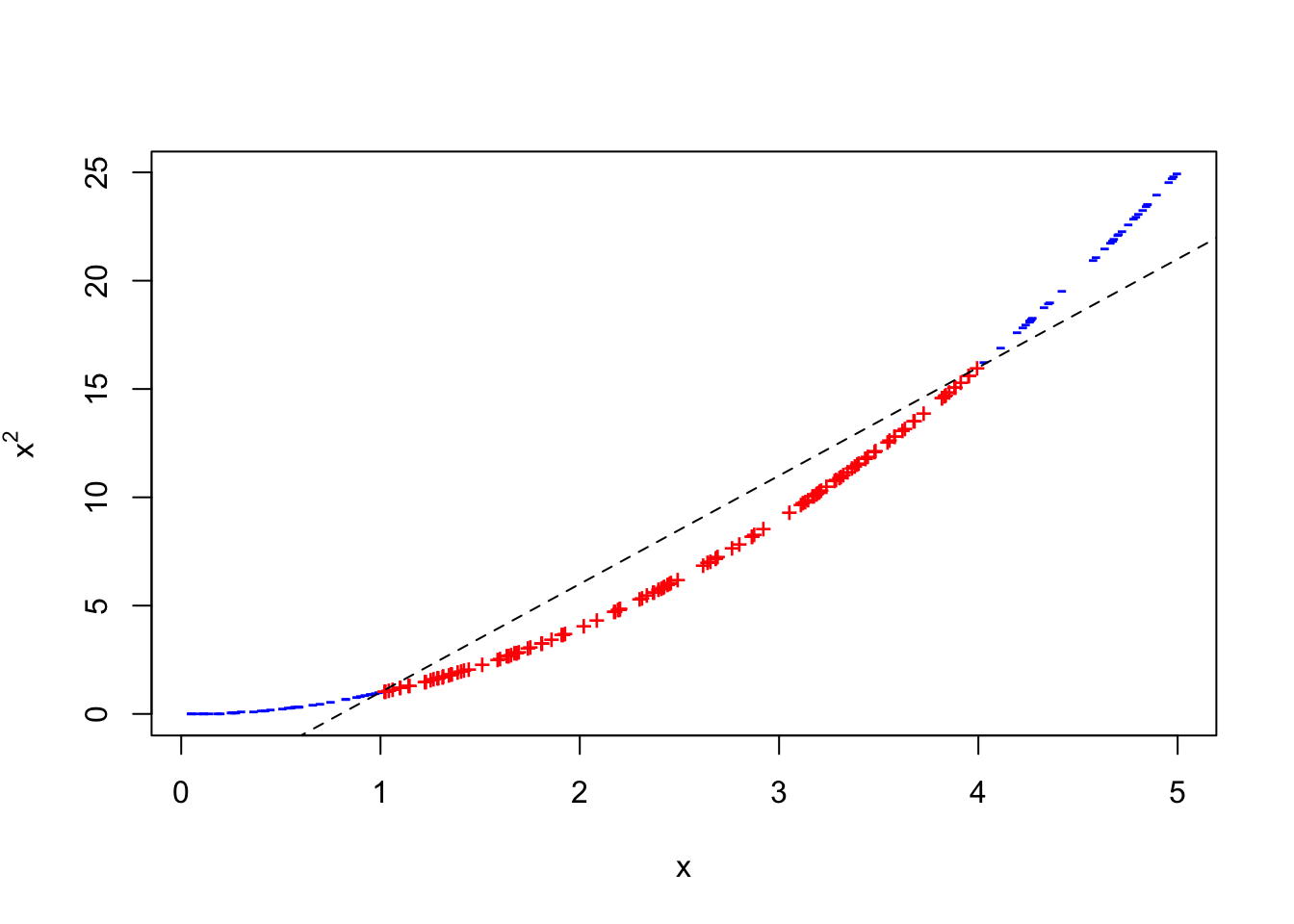The moral we derive from these examples (and from many others like them) is that, in order to predict well, we’d like to make use of lots and lots of nonlinear features. But we would also like to calculate quickly, and to not overfit all the time, and both of these are hard when there are many features.

Support vector machines are ways of getting the advantages of many nonlinear features without some of the pain pains. They rest on three ideas: the dual representation of linear classifiers; the kernel trick; and margin bounds on generalization. The dual representation is a way of writing a linear classifier not in terms of weights over features, $$w_j$$, $$j\in 1:p$$, but rather in terms of weights over training vectors, $$\alpha_i$$, $$i \in 1:n$$. The kernel trick is a way of implicitly using many, even infinitely many, new, nonlinear features without actually having to calculate them. Finally, margin bounds guarantee that kernel-based classifiers with large margins will continue to classify with low error on new data, and so give as an excuse for optimizing the margin, which is easy.

Notation Input consists of $$p$$-dimensional vectors $$\vec{X}$$. We’ll consider just two classes, $$Y=+1$$ and $$Y=-1$$.

# Dual Representation and Support Vectors

Recall that a linear classifier predicts $$\hat{Y}(\vec{x}) = \Indicator{b+\vec{x}\cdot\vec{w} \geq 0}$$. That is, it hopes that the data can be separated by the plane with normal (perpendicular) vector $$\vec{w}$$, offset a distance $$b$$ from the origin. We have been looking at the problem of learning linear classifiers as the problem of selecting good weights $$\vec{w}$$ for input features. This is called the primal representation, and we’ve seen several ways to do it — the prototype method, logistic regression, etc.

The weights $$\vec{w}$$ in the primal representation are weights on the features, and functions of the training vectors $$\vec{x}_i$$. A dual representation gives weights to the training vectors. That is, the classifier predicts $\hat{Y}(\vec{x}) = \Indicator{b + \sum_{i=1}^{n}{\alpha_i \left(\vec{x}_i \cdot \vec{x}\right)} \geq 0}$ where $$\alpha_i$$ are now weights over the training data. We can always find such dual representations when $$\vec{w}$$ is a linear function of the vectors, as in the prototype method6 or the perceptron algorithm7, or if we have more data points than features8. But we could also search for those weights directly. We would typically expect $$\alpha_i$$ to be $$> 0$$ if $$i$$ is in the positive class, and $$< 0$$ if $$i$$ is in the negative class. It’s sometimes convenient to incorporate this into the definition, so that we say $\hat{Z}(\vec{x}) = \sgn{\left(b + \sum_{i=1}^{n}{\alpha_i z_i \left( \vec{x}_i \cdot \vec{x}\right)}\right)}$ and insist that $$\alpha_i > 0$$.

There are a couple of things to notice about dual representations.

1. We need to learn the $$n$$ weights in $$\vec{\alpha}$$, not the $$p$$ weights in $$\vec{w}$$. This can help when $$p \gg n$$.
2. The training vector $$\vec{x}_i$$ appears in the prediction function only in the form of its inner product with the text vector $$\vec{x}$$, $$\vec{x}_i \cdot \vec{x} = \sum_{j=1}^{p}{x_{ij} x_j}$$.
3. We can have $$\alpha_i = 0$$ for some $$i$$. If $$\alpha_i \neq 0$$, then $$\vec{x}_i$$ is a support vector. The fewer support vectors there are, the more sparse the solution is.

The first two attributes of the dual representation play in to the kernel trick. The third, unsurprisingly, turns up in the support vector machine.

# The Kernel Trick

I’ve mentioned several times that linear models can get more power if instead of working directly with the input features $$\vec{x}$$, one first calculates new, nonlinear features $$\phi_1(\vec{x}), \phi_2(\vec{x}), \ldots \phi_q(\vec{x})$$ from the input. Together, these features form a vector, $$\phi(\vec{x})$$. One then uses linear methods on the derived feature-vector $$\phi(\vec{x})$$. To do polynomial classification, for example, we’d make the functions all the powers and combinations of powers of the input features up to some maximum order $$d$$, which would involve $$q = {p+d \choose d}$$ derived features. Once we have them, though, we can do linear classification in terms of the new features.

There are three difficulties with this approach; the kernel trick solves two of them.

1. We need to construct useful features.
2. The number of features may be very large. (With order-$$d$$ polynomials, the number of features goes roughly as $$d^p$$.) Even just calculating all the new features can take a long time, as can doing anything with them.
3. In the primal representation, each derived feature has a new weight we need to estimate, so we seem doomed to over-fit.

The only thing to be done for (1) is to actually study the problem at hand, use what’s known about it, and experiment. Items (2) and (3) however have a computational solution.

Remember, in the dual representation, training vectors only appear via their inner products with the test vector. If we are working with the new features, this means that the classifier can be written $\begin{eqnarray} \hat{Z}(\vec{x}_0) & = & \sgn{\left(b + \sum_{i=1}^{n}{\alpha_i z_i \phi(\vec{x}_i) \cdot \phi(\vec{x}_0)} \right)}\\ & = & \sgn{\left(b + \sum_{i=1}^{n}{\alpha_i z_i \sum_{j=1}^{q}{\phi_j(\vec{x_i}) \phi_j(\vec{x}_0)}} \right)}\\ & = & \sgn{\left(b + \sum_{i=1}^{n}{\alpha_i z_i K_{\phi}(\vec{x}_i,\vec{x}_0)} \right)} \label{eqn:kernel-classifier} \end{eqnarray}$ where the last line defines $$K$$, a (nonlinear) function of $$\vec{x}_i$$ and $$\vec{x}$$: $\begin{equation} K_{\phi}(\vec{x}_i,\vec{x}) \equiv \sum_{j=1}^{q}{\phi_j(\vec{x_i}) \phi_j(\vec{x})} \end{equation}$ $$K_{\phi}$$ is the kernel9 corresponding to the features $$\phi$$.

Any classifier of the form $\hat{Z}(\vec{x}_0) = \sgn{\left(b + \sum_{i=1}^{n}{\alpha_i z_i K_{\phi}(\vec{x}_i, \vec{x}_0)} \right)}$ is a kernel classifier.

The thing to notice about kernel classifiers is that the actual features matter for the prediction only to the extent that they go into computing the kernel $$K_{\phi}$$. If we can find a short-cut to get $$K_{\phi}$$ without computing all the features, we don’t actually need the features.

To see that this is possible, consider the expression $$(\vec{x}\cdot\vec{z} +1/\sqrt{2})^2 - 1/2$$. A little algebra shows that $(\vec{x}\cdot\vec{z} +1/\sqrt{2})^2 - \frac{1}{2}= \sum_{j=1}^{p}{\sum_{k=1}^{p}{(x_j x_k) (z_j z_k)}} + \sum_{j=1}^{p}{x_j z_j}$ This says that the left-hand side is the kernel for the $$\phi$$ which maps the input features to all quadratic (second-order polynomial) functions of the input features. By taking $$(\vec{x}\cdot\vec{z} + c)^d$$, we can evaluate the kernel for polynomials of order $$d$$, without having to actually compute all the polynomials. (Changing the constant $$c$$ changes the weights assigned to higher-order versus lower-order derived features.)

In fact, we do not even have to define the features explicitly. The kernel is the dot product (a.k.a. inner product) on the derived feature space, which says how similar two feature vectors are. We really only care about similarities, so we can get away with any function $$K$$ which is a reasonable similarity measure. The following theorem will not be proved here, but justifies just thinking about the kernel, and leaving the features implicit.

Mercer’s Theorem If $$K_{\phi}(\vec{x},\vec{z})$$ is the kernel for a feature mapping $$\phi$$, then for any finite set of vectors $$\vec{x}_1, \ldots \vec{x}_m$$, the $$m \times m$$ matrix $$K_{ij} = K_{\phi}(\vec{x}_i, \vec{x}_j)$$ is symmetric, and all its eigenvalues are non-negative. Conversely, if for any set of vectors $$\vec{x}_1, \ldots \vec{x}_m$$, the matrix formed from $$K(\vec{x}_i, \vec{x}_j)$$ is symmetric and has non-negative eigenvalues, then there is some feature mapping $$\phi$$ for which $$K(\vec{x}, \vec{z}) = \phi(\vec{x})\cdot\phi(\vec{z})$$.

So long as a kernel function $$K$$ behaves like an inner product should, it is an inner product on some feature space, albeit possibly a weird one. (Sometimes the feature space guaranteed by Mercer’s theorem is an infinite-dimensional one.) The moral is thus to worry about $$K$$, rather than $$\phi$$. Insight into the problem and background knowledge should go into building the kernel. The fundamental job of the kernel is to say how similar two different data points are;

This can be simplified by the fact (which we also will not prove) that sums and products of kernel functions are also kernel functions.

### An Example: The Gaussian/Radial Kernel

The Gaussian density function $$\frac{1}{\sqrt{2\pi\sigma^2}}e^{-\|\vec{x}-\vec{x}\|^2/2\sigma^2}$$ is a valid kernel. In fact, from the series expansion $$e^u = \sum_{n=0}^{\infty}{\frac{u^n}{n!}}$$, we can see that the implicit feature space of the Gaussian kernel includes polynomials of all orders. This means that even though we’re just using one kernel function, we’re implicitly using infinitely many transformed features!

When working with kernel methods, we typically10 write $$K(\vec{x},\vec{z}) = \exp{-\gamma \|\vec{x}-\vec{z}\|^2}$$, so that the normalizing constant of the Gaussian density is absorbed into the dual weights, and $$\gamma = 1/2\sigma^2$$. This is sometimes also called the radial (or radial basis) kernel. The scale factor $$\gamma$$ is a control setting. A typical default is $$\gamma$$ is the median of $$\|\vec{x}_i - \vec{x}_j\|^2$$ over the training data (after first scaling the raw features to unit variance). If you are worried that it matters, you can always tune it by cross-validation.

## Kernelization

The advantages of the kernel trick are that:

1. we get to implicitly use many nonlinear features of the data, without wasting time having to compute them; and
2. by combining the kernel with the dual representation, we need to learn only $$n$$ weights, rather than one weight for each new feature. We can even hope that the weights are sparse, so that we really only have to learn a few of them.

Closely examining linear regression models shows that almost everything they do with training vectors involves only inner products, $$\vec{x}_i \cdot \vec{x}$$ or $$\vec{x}_i \cdot \vec{x}_j$$. These inner products can be replaced by kernels, $$K(\vec{x}_i, \vec{x})$$ or $$K(\vec{x}_i, \vec{x}_j)$$. Making this substitution throughout gives the kernelized version of the linear procedure. Thus in addition to kernel classifiers (= kernelized linear classifiers), there is kernelized regression, kernelized principal components, etc. For instance, in kernelized regression, we try to approximate the conditional mean by a function of the form $$\sum_{i=1}^{n}{\alpha_i K(\vec{x}_i,\vec{x})}$$, where we pick $$\alpha_i$$ to minimize the residual sum of squares. In the interest of time I am not going to follow through any of the math.

# Margin Bounds

To recap, once we fix a kernel function $$K$$, our kernel classifier has the form $\hat{Z}(\vec{x}) = \sgn{\left(b + \sum_{i=1}^{n}{\alpha_i z_i K(\vec{x}_i,\vec{x})}\right)}$ and the learning problem is just finding the $$n$$ dual weights $$\alpha_i$$ and the off-set $$b$$. There are several ways we could do this.

1. Kernelize/steal a linear algorithm: Take any learning procedure for linear classifiers; write it so it only involves inner products; then run it, substituting $$K$$ for the inner product throughout. This would give us a kernelized perceptron algorithm, for instance.
2. Direct optimization: Treat the in-sample error rate, or maybe the cross-validated error rate, as an objective function, and try to optimize the $$\alpha_i$$ by means of some general-purpose optimization method. This is tricky, since the indicator function means that the error rate depend discontinuously on the $$\alpha$$’s, and changing predictions on one training point may mess up other points. This sort of discrete, inter-dependent optimization problem is generically very hard, and best avoided.
3. Optimize something else: Since what we really care about is the generalization to new data, find a formula which tells us how well the classifier will generalize, and optimize that. Or, less ambitiously, find a formula which puts an upper bound on the generalization error, and make that upper bound as small as possible.

The margin-bounds idea consists of variations on the third approach.

Recall that for a classifier, the margin of data-point $$i$$ is its distance to the boundary of the classifier. For a (primal-form) linear classifier $$(b, \vec{w})$$, this is $\gamma_i = z_i \left(\frac{b}{\|\vec{w}\|} + \vec{x}_i \cdot \frac{\vec{w}}{\|\vec{w}\|}\right)$ This quantity is positive if the point is correctly classified. It shows the “margin of safety” in the classification, i.e., how far the input vector would have to move before the predicted classification flipped. The over-all margin of the classifier is $$\gamma = \min_{i \in 1:n}{\gamma_i}$$.

## Generalization error for classifiers with zero in-sample error in terms of margin

Large-margin linear classifiers tend to generalize well to new data (from the same source). The basic reason is that there just aren’t many linear surfaces which manage to separate the classes with a large margin. As we demand a higher and higher margin, the range of linear surfaces that can deliver it shrinks. The margin thus effectively controls the capacity for over-fitting: high margin means small capacity, and low risk of over-fitting. I will now quote three specific results for linear classifiers, presented without proof11.

Margin bound for perfect separation (Cristianini and Shawe-Taylor 2000, Theorem 4.18): Suppose the data come from a distribution where $$\|\vec{X}\| \leq R$$. Fix any number $$\gamma$$ > 0. If a linear classifier correct classifies all $$n$$ training examples, with a margin of at least $$\gamma$$, then with probability at least $$1-\delta$$, its error rate on new data from the same distribution is at most $\varepsilon = \frac{2}{n}\left( \frac{64R^2}{\gamma^2}\ln{\frac{en\gamma}{8 R^2}\ln{\frac{32n}{\gamma^2}}} + \ln{\frac{4}{\delta}}\right)$ if $$n > \min{64R^2/\gamma^2, 2/\varepsilon}$$.

Notice that the promised error rate gets larger and larger as the margin shrinks. This suggests that what we want to do is maximize the margin (since $$R^2$$, $$n$$, $$64$$, etc., are beyond our control).

## Classifiers with non-zero in-sample error: “soft margin” and “slack”

Suppose we can’t find a perfect classifier; we can still want to

The next result applies to imperfect classifiers. Fix a minimum margin $$\gamma_0$$, and define the slack $$\zeta_i$$ of each data point as $h_i(\gamma_0) = \max{\left\{0, \gamma_0 - \gamma_i\right\}}$ That is, the slack is the amount by which the margin falls short of $$\gamma_0$$, if it does. If the separation is imperfect, some of the $$\gamma_i$$ will be negative, and the slack variables at those points will be $$> \gamma_0$$. In particular, we can say that a classifier “has margin $$\gamma$$ with slacks $$h_1, \ldots h_n$$” if the minimum margin on the correctly classified points is $$\gamma$$, and we use that in to calculate the slacks needed to accommodate the mis-classified points.

Soft margin bound/slack bound for imperfect separation (Cristianini and Shawe-Taylor 2000, Theorem 4.22): Suppose a linear classifier achieves a margin $$\gamma$$ on $$n$$ samples, if allowed slacks $$\vec{h}(\gamma) = (h_1(\gamma), h_2(\gamma), \ldots h_n(\gamma))$$. Then there’s a constant $$c> 0$$ such that, with probability at least $$1-\delta$$, its error rate on new data from the same source is at most $\varepsilon = \frac{c}{n}\left(\frac{R^2 + \|\vec{h}(\gamma)\|^2}{\gamma^2}\ln^2{n} - \ln{\delta}\right)$

(Notice that if we can set all the slacks to zero, because there’s perfect classification with some positive margin, then we get a bound that looks like, but isn’t quite, the perfect-separation bound again.)

This suggests that the quantity to optimize is the ratio $$\frac{R^2 + \|\vec{h}\|^2}{\gamma^2}$$. There is a trade-off here: adjusting the boundary to move it away from points we already correctly classify will increase $$\gamma$$, but usually at the cost of increasing the slacks (and so increasing $$\|\vec{h}\|^2$$). It could even move the boundary enough so that a point we used to get right is now mis-classified, moving one of the slacks from zero to positive.

## Hinge loss

The notion of hinge loss is closely related to that of slack. If our classifier is $\hat{Z}(\vec{x}) = \sgn{t(\vec{x})}$ e.g., $$t(\vec{x}) = b + \sum_{i=1}^{n}{\alpha_i z_i \left(\vec{x}_i \cdot \vec{x}\right)}$$, then the hinge loss at the point $$\vec{x}_i, z_i$$ is $h_i = \max{\left\{ 0, 1 - z_i t(\vec{x}_i)\right\}}$ To see what this does, look at a plot, say for when $$Y=Z=1$$.